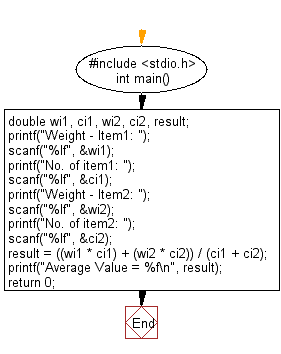﻿ C Program: Calculate the average value of the items - w3resource

# C Exercises: Calculate the average value of the items

## C Basic Declarations and Expressions: Exercise-11 with Solution

Write a C program that accepts two item's weight and number of purchases (floating point values) and calculates their average value.

C Code:

``````#include <stdio.h>

int main()
{
double wi1, ci1, wi2, ci2, result; // Declare variables for weights and counts

// Prompt user for weight and count of item 1
printf("Weight - Item1: ");
scanf("%lf", &wi1);

// Prompt user for count of item 1
printf("No. of item1: ");
scanf("%lf", &ci1);

// Prompt user for weight and count of item 2
printf("Weight - Item2: ");
scanf("%lf", &wi2);

// Prompt user for count of item 2
printf("No. of item2: ");
scanf("%lf", &ci2);

// Calculate average value
result = ((wi1 * ci1) + (wi2 * ci2)) / (ci1 + ci2);

// Print the average value
printf("Average Value = %f\n", result);

return 0;
}

``````

Sample Output:

```Weight - Item1: 15
No. of item1: 5
Weight - Item2: 25
No. of item2: 4
Average Value = 19.444444
```

Flowchart:C Programming Code Editor:

What is the difficulty level of this exercise?

Test your Programming skills with w3resource's quiz.

﻿